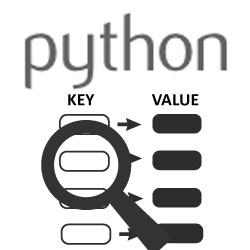# Python : How to check if any element of a list is a key in dictionaryRecently, while I was working on a Python script, I had a dictionary and a list of string elements and I needed to check if any of the element in that list was also used as a key in the dictionary. This article will show you two solutions for this problem. One will simply use iteration of a for loop, while the other will use Python sets.

For our example, let's first create a list of strings and a dictionary with some random numbers as their values.

```my_list=["A", "B", "C", "D"]
my_dict={"ABC":"10", "MM":"3", "A":"8"}
```

So the question is, how to check if any element in my_list also appears in a dictionary as a key in my_dict.

## Solution 1 - iterating through the list

Here, we simply iterate through each element in the list. In each iteration, we check if the element exists as a key in the dictionary (line 2). When the key is found, we do what we need to do and then break from the loop. If we need to find all the elements used as a dictionary key, we simply remove the `break` on line 4.

```for e in my_list:
if e in my_dict:
print("At least one element was found. Do something.")
break
```

This solution is straight forward, but in my script, the list elements would rarely be found as keys in the dictionary, so the use of loops seemed wasteful to me and I wanted to try to use a solution without the `for` loop.

## Solution 2 - making use of sets

For this solution, we make use of Python built-in set types. Sets are unordered collection of elements where each element is unique. In the code below, we convert both the list and the dictionary into sets. The set from the dictionary my_dict_set will contain the keys of the dictionary, while the set from the list my_list_set will contain unique elements of the list.

Next, we use the set intersection() method that will return a new set containing elements that exist in both of the my_dict_set and my_list_set sets. If there is at least one element used as a key of the dictionary, the `if` statement in line 3 returns true, so the `if` block gets executed (line 4).

```my_list_set=set(my_list)
my_dict_set=set(my_dict)
if my_list_set.intersection(my_dict_set):
print("At least one element was found. Do something.")
```

If you need to loop through all the elements that are used as the dictionary key, we replace the `if` statement with the loop as shown here:

```for i in my_list_set.intersection(my_dict_set):
print("Element "+i+" was found. Do something.")
```
Note: When `intersection()` method doesn't find any elements that are in both sets, it returns an empty `set()` and not `None`.

## Conclusion

In this article, we show two ways to check if any element in the list of strings is also used as a key in a dictionary. First, we used a `for` loop and for the second solution, we used the Python built-in `sets` type.

Did you have to solve a similar problem and used a different solution? Let us know and we might add it here.